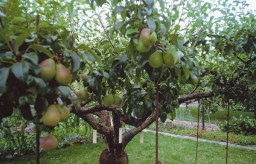# Cherries 19903

There are pears, apples, and cherries in the alley. There are a total of 1,075 trees. Pear is twice as much as apple, and cherry is 30% more than apple. How many trees are there?

h =  500
j =  250
v =  325

### Step-by-step explanation:

h+j+v=1075
h=2j
v = j + 0.30j

h+j+v=1075
h=2·j
v = j + 0.30·j

h+j+v = 1075
h-2j = 0
1.3j-v = 0

Row 2 - Row 1 → Row 2
h+j+v = 1075
-3j-v = -1075
1.3j-v = 0

Row 3 - 1.3/-3 · Row 2 → Row 3
h+j+v = 1075
-3j-v = -1075
-1.433v = -465.833

v = -465.83333333/-1.43333333 = 325
j = -1075+v/-3 = -1075+325/-3 = 250
h = 1075-j-v = 1075-250-325 = 500

h = 500
j = 250
v = 325

Our linear equations calculator calculates it.Did you find an error or inaccuracy? Feel free to write us. Thank you!

Tips for related online calculators